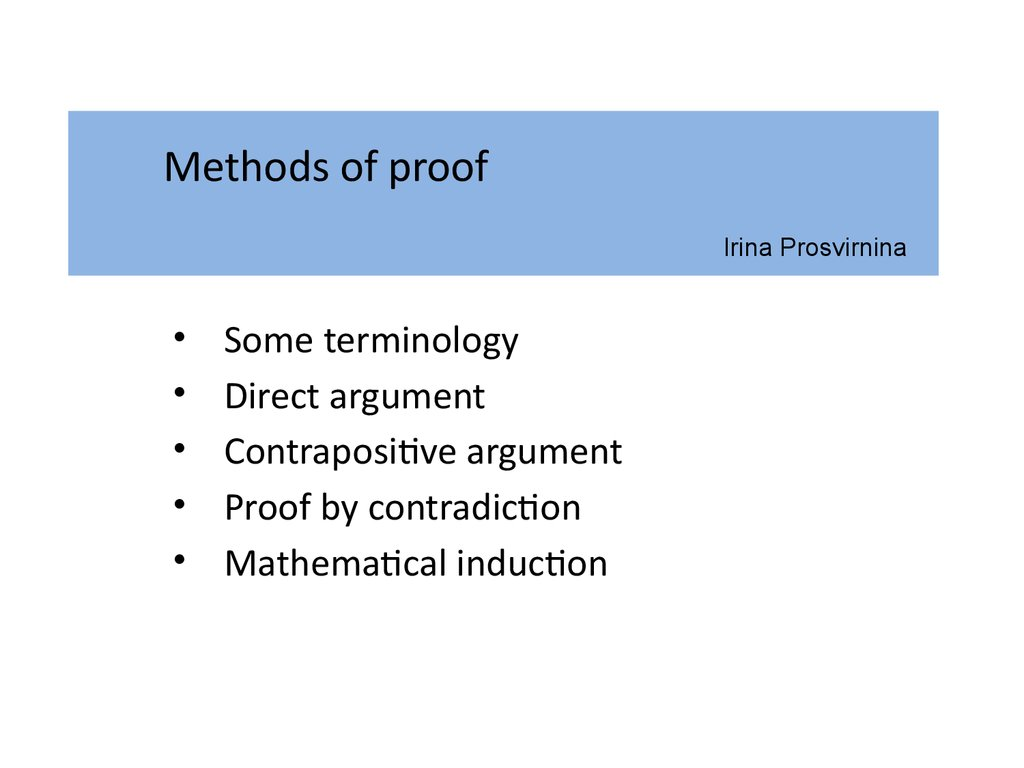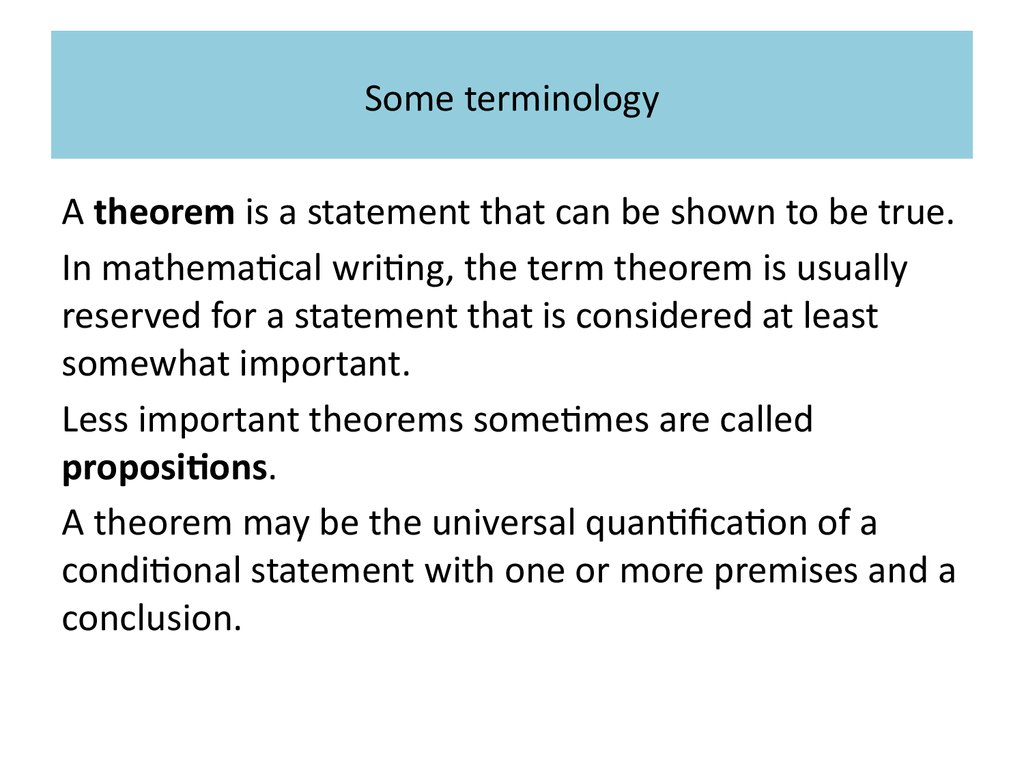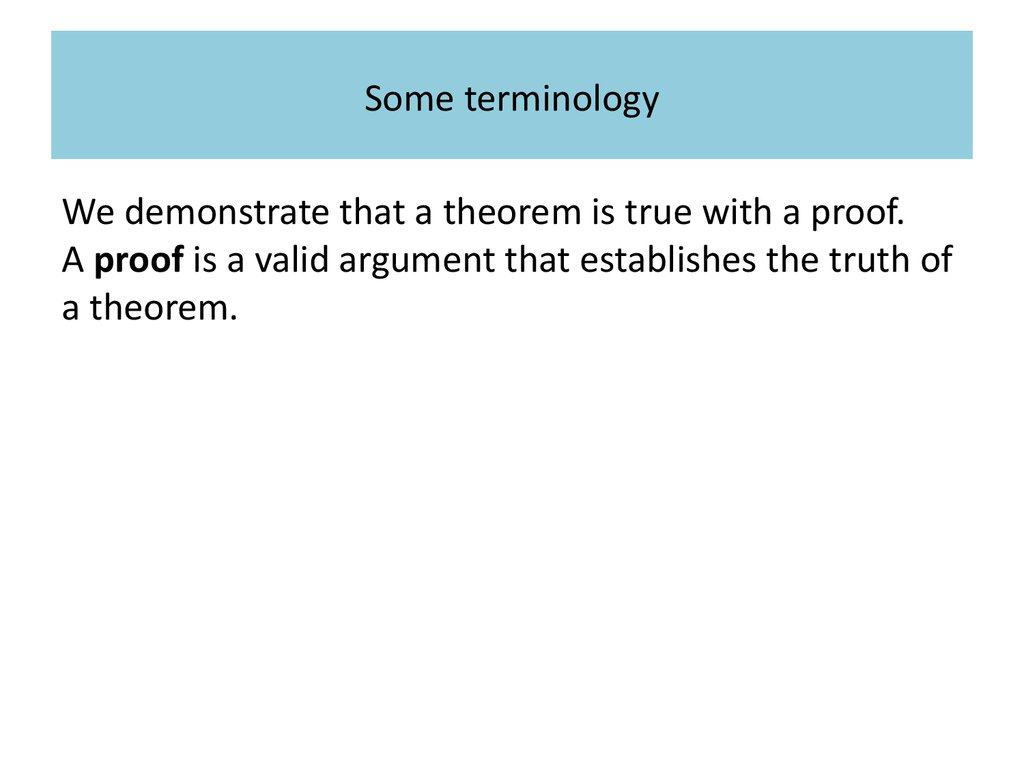# Methods of proof

## 1. Methods of proof Irina Prosvirnina

Some terminology
Direct argument
Contrapositive argument
Mathematical induction

## 2. Some terminology

A theorem is a statement that can be shown to be true.
In mathematical writing, the term theorem is usually
reserved for a statement that is considered at least
somewhat important.
Less important theorems sometimes are called
propositions.
A theorem may be the universal quantification of a
conditional statement with one or more premises and a
conclusion.

## 3. Терминология

Some
Терминология
terminology
We demonstrate that a theorem is true with a proof.
A proof is a valid argument that establishes the truth of
a theorem.

## 4. Some terminology

The statements used in a proof can include
axioms (or postulates), which are statements we
assume to be true,
the premises, if any, of the theorem,
and previously proven theorems.

## 5. Some terminology

Axioms may be stated using primitive terms that do not
require definition, but all other terms used in theorems
and their proofs must be defined.

## 6. Some terminology

Rules of inference, together with definitions of terms,
are used to draw conclusions from other assertions,
tying together the steps of a proof. In practice, the final
step of a proof is usually just the conclusion of the
theorem.

## 7. Some terminology

A less important theorem that is helpful in the proof of
other results is called a lemma (plural: lemmas or
lemmata).
Complicated proofs are usually easier to understand
when they are proved using a series of lemmas, where
each lemma is proved individually.

## 8. Some terminology

A corollary is a theorem that can be established directly
from a theorem that has been proved.

## 9. Some terminology

A conjecture is a statement that is being proposed to
be a true statement, usually on the basis of some
partial evidence, a heuristic argument, or the intuition
of an expert.
When a proof of a conjecture is found, the conjecture
becomes a theorem. Many times conjectures are
shown to be false, so they are not theorems.

## 10. Methods of proof

In practice, the proofs of theorems designed for human
consumption are almost always informal proofs,
where more than one rule of inference may be used
in each step, where steps may be skipped,
where the axioms being assumed and the rules of
inference used are not explicitly stated.

## 11. Methods of proof

Informal proofs can often explain to humans why
theorems are true, while computers are perfectly
happy producing formal proofs using automated
reasoning systems.

## 12. Methods of proof

The methods of proof discussed here are important not
only because they are used to prove mathematical
theorems, but also for their many applications to
computer science.
These applications include
verifying that computer programs are correct,
establishing that operating systems are secure,
making inferences in artificial intelligence,
showing that system specifications are consistent,
and so on.

## 13. Methods of proof

Consequently, understanding the techniques used in
proofs is essential both in mathematics and in
computer science.

## 14. Methods of proof

•Logical arguments are used to give us proofs of the
theorems.
In computing such proofs are essential in the design
and verification of algorithms.
The commonest types of proof are ones where we wish
to establish the truth of a proposition of the form
.

## 15. Methods of proof

There are several standard methods of proof, including
the following:
direct argument,
contrapositive argument,

## 16. Direct argument

•1. Direct argument
Assume is true and show that is true. This rules out
the situation where is true and is false which is the
only case where is false.

## 17. Contrapositive argument

•2. Contrapositive argument
Assume is false and show that is false. This
demonstrates that
is true which is the same as showing that
is true.

Assume is true and is false and derive a
contradiction. This again rules out the situation where
is true and is false which is the only case where
is false.

## 19. Example 1 Use a direct method of proof to show that if х and у are odd integers, then ху is also odd.

•Solution
First, notice that if x is an odd integer then х = 2т + 1,
where т is an integer.Similarly, у = 2n + 1 for some
integer n.
Then,
ху = (2m + 1)(2n + 1)=
= 4mn + 2m + 2 + 1 =
= 2(2mn + m + n) + 1
Is an odd integer.

## 20. Example 2 Let n be a positive integer. Prove, using the contrapositive, that if n2 is odd, then n is odd.

•Solution
The negation of n2 is odd is n2 is even, and the negation
of n is odd is n is even. Therefore, we proof directly that
if n is even then n2 is even
Since n is even, we can write n = 2m for some integer
m. Then, n2 = 4m2 = 2(2m2) is also even.

## 21. Example 3 Use a proof by contradiction to show that if x2 = 2 then x is not a fraction.

Solution
By way of contradiction, assume that х is a fraction and
write х = m/n where n and m are integers, n is not equal
to 0 and n and m have no common factors. Since x2 = 2,
we have that (m/n)2 = 2. Therefore, m2 = 2 n2. But this
implies that m2 is an even integer. Therefore, т is an
even integer. Hence, т = 2р for some other integer р.

## 22. Example 3 Use a proof by contradiction to show that if x2 = 2 then x is not a fraction.

•Solution
Substituting this information into the equation
m2 = 2 n2 leads to 4 p2 = 2 n2, n2 = 2 p2. But then, n is
also an even integer. We have shown that m and n have
a common factor (of 2) which contradicts our original
assertion that m and n have no common factors.
This contradiction can only be resolved by concluding
that if x2 = 2 then x is not a fraction.

## 23. Mathematical induction

In computing a program is said to be correct if it
behaves in accordance with its specification. Whereas
program testing shows that selected input values give
acceptable output values, proof of correctness uses
formal logic to prove that for any input values, the
output values are correct.
Proving the correctness of algorithms containing loops
requires a powerful method of proof called
mathematical induction.

## 24. Mathematical induction

Consider the following recursive algorithm, intended to
calculate the maximum element in a list a1, a2, …, an of
positive integers.
begin
г:=0;
М:=0;
while г < n do
begin
r :=r+1;
M:=max(M, ar);
end
еnd

## 25. Mathematical induction

To see how the algorithm works consider the input list
a1 = 4, a2 = 7, a3 = 3 and a4 = 8. The trace table is given in
the next table.
r
M
r<4?
0
0
Yes
1
4
Yes
2
7
Yes
3
7
Yes
4
8
No

## 26. Mathematical induction

r
0
1
2
3
4
M
0
4
7
7
8
r<4?
Yes
Yes
Yes
Yes
No
The output is М = 8, which is correct. Notice that after
each execution of the loop, М is the maximum of the
elements of the list so far considered.

## 27. Mathematical induction

So does the algorithm for all lists of any length n?
Consider an input a1, a2, …, an of length n and let Mk be
the value of М after k executions of the loop.
1) For an input list a1 of length 1, the loop is executed
once and M is assigned to be the maximum of 0 and
a1,which is just a1. It is the correct input.
2) If after k executions of the loop, Mk is the maximum
element of the list a1, a2, …, ak then after one more
loop Mk+1 is assigned the value max(Mk, ak+1 ) which
will then be the maximum element of the list a1, a2,
…, ak+1.

## 28. Mathematical induction

By condition 1) the algorithm works for any list of
length 1, and so by condition 2) it works for any list of
length 2. By condition 2) again it works for any list of
length 3, and so on. Hence, the algorithm works for any
list of length n and so the algorithm is correct.
This process can be formalised as follows.

## 29. Mathematical induction

The principle of mathematical induction
Let Р(n) be a predicate that is defined for all natural n.
Suppose that
1) Р(1) is true and
2) For all 1
(Р(к) Р(k+1)) is true.
Then Р(n) is true for all n 1.

## 30. Mathematical induction

•Example 1 Prove, by induction, that for all n 1
1+ 2 + … + n = n(n+1)/2.
Solution
Let Р(n) be the predicate 1+ 2 + … + n = n(n+1)/2.
In the case n = 1, the left-hand side is simply 1, and the
right-hand side is 1(1+1)/2 = 1.
Therefore, Р(1) is true.

## 31. Mathematical induction

•Assume now that
1+ 2 + … + k = k(k+1)/2 for some k 1. Then
1+ 2 + … + (k+1) = (1+ 2 + … +k) + (k+1)
= k(k+1)/2 + (k+1)
= (k(k+1) + 2(k+1))/2
= ((k+2)(k+1))/2
= (k+1)(k+2)/2 .
Hence, for all k 1(Р(k) Р(k+1)) is true. Therefore, by
induction Р(n) is true for all n 1.

## 32. Mathematical induction

•Example 2 Prove, by induction, that 7n – 1 is divisible by
6 for all n 1.
Solution
First, note that an integer a is divisible by an integer b if
there is some other integer m with
а = mb.
For example, 51 is divisible by 17 since 51 = 3 • 17.
Let Р(n) be the predicate «7n – 1 is divisible by 6».
In the case n = 1,
7n – 1 = 71 – 1 = 7 – 1 = 6,
which is clearly divisible by 6. Therefore, Р(1) is true.

## 33. Mathematical induction

•Assume now that 7k – 1 is divisible by 6 for some k 1.
Then,
7k+1 – 1 = 7(7k) – 1
= 7(7k – 1) + 7 – 1
= 7(7k – 1) + 6 .
Since 7k – 1 is divisible by 6 it follows that 7(7k – 1) + 6 is
also is divisible by 6.
Hence, 7k+1 – 1 is divisible by 6 and so (Р(k) Р(k+1)) for
all k 1 is true.
Therefore, by induction Р(n) is true for all n 1.

## 34. Mathematical induction

Example 3
A sequence of integers x1, x2, …, xn is defined recursively
as follows:
x1 = 1 and xk+1 = xk + 8k for к >= 1.
Prove that
xn = (2n – 1)2 for all n >= 1.
Solution
Let Р(n) be the predicate xn = (2n – 1)2. In the case n = 1,
(2n – 1)2 = (2 • 1 – 1)2 = 1. Therefore, Р(1) is true.

## 35. Mathematical induction

•Assume now that xk = (2k – 1)2 for some k 1.
Then
xk+1 = xk + 8k
= (2k – 1)2 + 8k
= 4k2 + 4k + 1
= (2k + 1)2 .
Hence,
xk+1 = (2(k + 1) – 1)2 .
And so (Р(k) Р(k+1)) is true for all k 1. Therefore, by
induction Р(n) is true for all n 1.